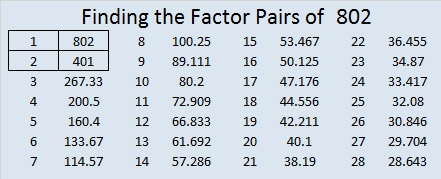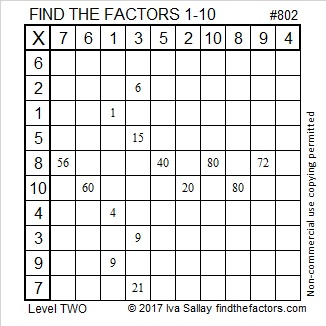# 802 Pi Day at Smith’s

In the United States tomorrow’s date is written 3-14. Because 3.14 is a famous approximation for π (pi), people all over the country will eat pie to celebrate Pi Day. This afternoon I took a picture of this sign and the pie display at my local Smith’s Food and Drug.I took that picture right when I walked into the store, but there were no pies on display for National Pi Day.About 15 minutes later I returned to the display to take another picture. Now there were pies on the table! I told a salesperson who I think worked on the display that I was going to take a picture and put it on my blog. She asked what kind of a blog I wrote. I told her a math blog. She looked puzzled and asked why I would want to put a picture of pies on a math blog. Then she turned around, looked at the display, and said something like, “Oh, now I get it, the number pi.”

How do you choose between apple, cherry, or peach pie? It’s much easier if you choose two and then you can get a free 8 oz. Cool Whip, too. Yummy.

If by chance you prefer pizza pi, here’s a thought from twitter that is often repeated in March:

And here’s some original artwork that displays pi in a way I had never thought of before:

BREAKING: secret of Pi revealed #PiDay pic.twitter.com/Ao8BQp31jd

You can also look here for a million digits of pi.

But pi is not the only interesting number in the world. Every number has its own curiosities. Let me tell you some reasons to get excited about the number 802:

802 is the sum of two squares:

• 21² + 19² = 802

So 802 is the hypotenuse of a Pythagorean triple:

• 80-798-802, which is 2 times another triple: 40-399-401.

It also means something else: Since odd numbers 21 and 19 have no common prime factors, 802 can be evenly divide by 2. Duh. . ., but it also means that unless 802 is also divisible by 5, 13, or 17, its only factors will be 2 and a prime number! Why are those three numbers the only ones I care about? Because they are the only prime number Pythagorean triple hypotenuses less than √802 ≈ 28.3.

Guess what? 5, 13, and 17 do not divide evenly into 802, so 802 is the product of 2 and a prime number which happens to be 401.

• 802 is a composite number.
• Prime factorization: 802 = 2 x 401
• The exponents in the prime factorization are 1 and 1. Adding one to each exponent and multiplying we get (1 + 1)(1 + 1) = 2 x 2 = 4. Therefore 802 has exactly 4 factors.
• Factors of 802: 1, 2, 401, 802
• Factor pairs: 802 = 1 x 802 or 2 x 401
• 802 has no square factors that allow its square root to be simplified. √802 ≈ 28.3196045Today’s puzzle is number 802 to distinguish it from every other puzzle I’ve made. Writing the numbers 1 – 10 in both the top row and the first column so that the factors and the clues work together as a multiplication table is as easy as pie!Print the puzzles or type the solution on this excel file: 10-factors 801-806

And here is a little more about the number 802:

802 is the sum of 8 consecutive prime numbers:

• 83 + 89 + 97 + 101 + 103 + 107 + 109 + 113 = 802

802 can also be written as the sum of three squares three different ways:

• 28² + 3² + 3² = 802
• 27² + 8² + 3² = 802
• 24² + 15² + 1² = 802

802 is also a palindrome in two other bases:

• 414 BASE 14 because 4(196) + 1(14) + 4(1) = 802
• 202 BASE 20 because 2(400) + 0(20) + 2(1) = 802